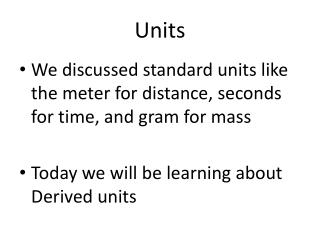DownloadDownload PresentationUnits

# Units

Download Presentation## Units

- - - - - - - - - - - - - - - - - - - - - - - - - - - E N D - - - - - - - - - - - - - - - - - - - - - - - - - - -
##### Presentation Transcript

1. Units • We discussed standard units like the meter for distance, seconds for time, and gram for mass • Today we will be learning about Derived units

2. Base Units and Derived Units?

3. Base Units vs. Derived Units • There are 7 Base units in the SI system Base units are independent of other units and only consist of one quantity. SI Base Units • Time – Seconds (s) • Length – Meter (m) • Mass – Kilogram (kg) • Temperature – Kelvin (K) • Amount of a substance – mole (mol) • Electric current – Ampere (A) • Luminous intensity – candela (cd)

4. Derived Units • A unit derived from a combination of base units is known as a derived unit. • Not all quantities can be measured with base units. • Speed for instance is measured in meters per second (m/s) :Note this includes two base units

5. Volume and Density – 2 derived units • Volume is space occupied by an object Measured in? Formula? • Density: the ratio which Compares the mass of an object to its volume Measured in? Formula?

6. Surface Area and Volume Problems • A box whose length is 5 cm, whose width is 2 cm and whose depth is 1 cm. What is the surface area? • A box whose length is 10 cm, whose width is 6 cm and whose depth is 2 cm. What is the surface area? • A dumpster is 10 meters in length, 4 meters in width and 2 meters in height. What is the volume of the dumpster? • Which room occupies more volume: the first room is 7 m long by 5 m wide; the second room is 8 m long by 4.5 m wide. Both rooms are 3 m in height.

7. Density Work Problems A chunk of paraffin (wax) has a mass of 50.4 grams and a volume of 57.9 cm3. What is the density of paraffin? A marble statue has a mass of 6,200 grams and a volume of 2,296 cm3. What is the density of marble?

8. 3. The density of cork is 0.24 g/cm3. What is the volume of a 240-gram piece of cork? 4. What is the mass of a pure platinum disk with a volume of 113 cm3? The density of platinum is 21.4 g/cm3. Give your answer in grams. 5. A cube made of an unknown material has a height of 9 cm. The mass of this cube is 3,645 grams. Calculate the density of this cube given this information?

9. 5. A student measured the string as 1.25 m long. The teacher said it was actually 2.12 m long. What was the student’s percent error? 6. The teacher said the volume of liquid was 500.0 mL. When measured, a student found it was 499.7 mL. What was the student’s percent error?

10. Slope of a Line?

11. Equation for Line Slope Find the slope of the line that goes through the points (-5, 3) and (2, 1). m= slope Y2 = second value on y axis Y1= first value on y axis X2= second value on the x axis X1= first value on the x axis

12. Slope Problems • Find the slope of a line with the following points: (4,2) and (5,6)? 2. Find the slope of the line that passes through the points (-1 , 0) and (3 , 8)? 3. Find the slope of the line that passes through the points (-2, -2) and (4, 1)?

13. Percent Error?

14. Error Absolute Error—how far the measured value is from the true value (positive number) Percent Error = x 100 Example: A student measures the density of a metal as 2.51 g/mL. The true density is 2.71 g/mL. What is the absolute error? What is the percent error?

15. Error Absolute Error—how far the measured value is from the true value (positive number) Percent Error = x 100 Example: A student measures the density of a metal as 2.51 g/mL. The true density is 2.71 g/mL. What is the absolute error? 2.51 – 2.71 =-0.21 g/mL = 0.21 g/mL What is the percent error? X 100 =7.7%

16. Percent Error Work Problems • Joshua uses his thermometer and finds the boiling point of ethyl alcohol to be 75 degrees C. He looks in a reference book and finds that the actual boiling point of ethyl alcohol is 80 degrees C. What is his percent error? • The density of water at 4oC is known to be 1.00 g/mL. Kayla experimentally found the density of water to be 1.075 g/mL. What is her percent error?

17. 3. The Handbook of Chemistry and Physics lists the density of a certain liquid to be 0.7988 g/mL. Taylor experimentally finds this liquid to have a density of 0.7925 g/mL. The teacher allows up to +/- 0.500% error to make an “A” on the lab. Did Fred make an “A”? Prove your answer. 4. An object has a mass of 35.0 grams. On Anthony’s balance, it weighs 34.85 grams. What is the percent error of his balance?